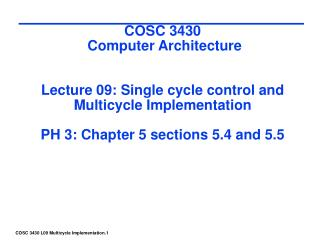DownloadDownload PresentationCOSC 3430 Computer Architecture Lecture 09: Single cycle control and Multicycle Implementation PH 3: Chapter 5 sectio

# COSC 3430 Computer Architecture Lecture 09: Single cycle control and Multicycle Implementation PH 3: Chapter 5 sectio

Télécharger la présentation## COSC 3430 Computer Architecture Lecture 09: Single cycle control and Multicycle Implementation PH 3: Chapter 5 sectio

- - - - - - - - - - - - - - - - - - - - - - - - - - - E N D - - - - - - - - - - - - - - - - - - - - - - - - - - -
##### Presentation Transcript

1. COSC 3430 Computer Architecture Lecture 09: Single cycle control and Multicycle ImplementationPH 3: Chapter 5 sections 5.4 and 5.5

2. Single cycle datapath control

3. Control • Selecting the operations to perform (ALU, read/write, etc.) • Controlling the flow of data (multiplexor inputs) • Information comes from the 32 bits of the instruction • Example: add \$8, \$17, \$18 • Instruction Format:000000 10001 10010 01000 00000 100000 op rs rt rd shamt func • ALU's operation based on instruction type and function code

4. Control • e.g., what should the ALU do with this instruction • Example: lw \$1, 100(\$2) • 35 2 1 100 op rs rt 16 bit offset • ALU control inputs as developed in B.6 0000 AND 0001 OR 0010 add 0110 subtract 0111 set-on-less-than 1100 NOR Not all of the above are used in this simplified datapath development

5. ALUOp is a 2 bit output computed from instruction type Control • Must describe hardware to compute 4-bit ALU control input from • given instruction type 00 = lw, sw 01 = beq, 10 = arithmetic • function code for arithmetic • Describe it using a truth table (can turn into gates):

6. Single cycle with control

7. Settings of the control lines from the opcode INSTRUCTION OPCODES Binary R-type 0 000000 Beq 4 000100 Lw 35 100011 Sw 43 101011

8. Control. Generation of the control signals from opcode

9. Truth table for the ALU 4 bit operation We show an implementation of this truth table with gates on the next slide. This could be considered a 3 bit output since the MSB is always 0 for our problem.

10. Control. Generating the 4 bit ALU operation from the ALUOp0 and ALUOp1 and the function code (bits 0-5) Example: Suppose ALUOP1 = 1 and F1 = 1 All others = 0 except ALUOP0 is X. Output should be 0110

11. Cycle 1 Cycle 2 Clk lw sw Waste Single Cycle Disadvantages & Advantages • Uses the clock cycle inefficiently – the clock cycle must be timed to accommodate the slowest instruction • especially problematic for more complex instructions like floating point multiply • May be wasteful of area since some functional units (e.g., adders) must be duplicated since they can not be shared during a clock cycle but • Is simple and easy to understand

12. Single Cycle Implementation (an example) • Calculate cycle time assuming negligible delays except: • memory (200ps), ALU and adders (100ps), register file access (50ps) • Assuming only the above delays, which of the following implementations would be faster and by how much? • An implementation in which every instruction operates in 1 clock cycle of a fixed length, or • An implementation where every instruction executes in 1 clock cycle using a variable-length clock, which for each instruction is only as long as it needs to be. (Such an approach is not practical, but it will allow us to see what is being sacrificed when all the instructions must execute in a single clock of the same length.)

13. Example continued • To compare performance, assume the following instruction mix: 25% loads, 10% stores, 45% ALU instructions, 15% branches, and 5% jumps. • First compare the CPU execution times using the equation • CPU time = Instr count × CPI × Clock cycle time, so • CPU time = IC × Clock cycle time, since CPI = 1 for both cases

14. Steps and times for various instructions

15. Example continued • The clock cycle for a machine with a single clock cycle time for all instructions will be determined by the longest instruction, which is 600ps, so CPU time = 600ps (IC). • A machine with a variable clock cycle time has an average time per instruction of CPU cycle = 600(25%) + 550(10%) + 400(45%) + 350(15%0 + 200(5%) = 447.5ps. • Since the variable clock has a shorter average clock cycle, it’s CPU time = 447.5ps (IC). The performance improvement is then 600/447.5 = 1.34.

16. Example continued • Hence the variable clock implementation is 1.34 times faster. • Unfortunately, implementing a variable speed clock for each instruction class is extremely difficult, and the overhead for such an approach could be larger than any advantage gained. As we will later see, an alternative is to use a shorter clock cycle that does less work and then vary the number of clock cycles for the different instruction classes. • The penalty for using a single-cycle design with a fixed clock cycle is significant, but might be acceptable for the small instruction set we are using. Early computers did exactly this. However, implementing a floating point unit for example, or an ISA with more complex instructions, wouldn’t work well at all.

17. Example continued • Because we must assume the clock cycle is equal to the worst-case delay for all instructions, we can’t use implementations that reduce the delay of the common case unless they also improve the worst case time. • A single cycle implementation thus violates one of our key design principles of making the common case fast.